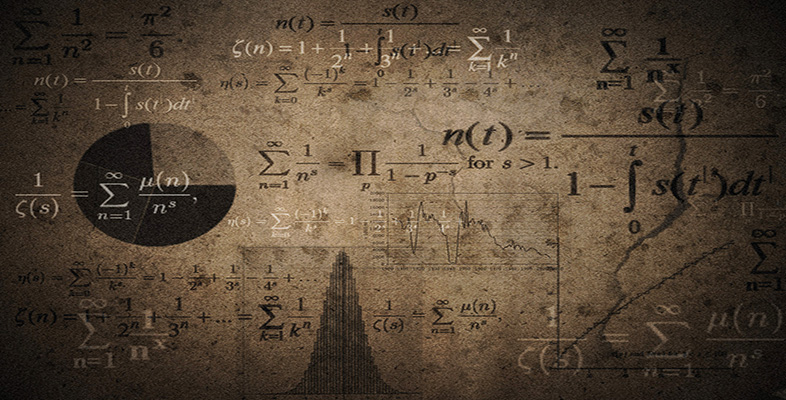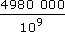Language, notation and formulas

This free course is available to start right now. Review the full course description and key learning outcomes and create an account and enrol if you want a free statement of participation.

Free course

# 6.2 Another ‘billions’ solution

Pretend that you are the marker of another solution to the same problem. How would you mark Solution B?

## Example 16 Billions

You may think that you know what the word billion means but do you really have a feel for its size?

 (a) Write down one billion as a power of 10.  (b) Consider how far a billion centimetres (cm) is. Determine what one billion cm is in kilometres (km). How does it compare with the distance between Saturn and the Sun, which is 1427000 000 km?  (c) Now consider one billion seconds. Determine how long this is in years (round your answer to the nearest whole number).  (d) Finally, if there are 4 980 000 grains in 1 kilogram (kg) of sugar, show that one billion grains of sugar are approximately equivalent to 2 × 102 kg of sugar. 

## Sample Solution B to Example 16

• (a) 109

• (b) 109 ÷ 102 ÷ 103 = 104

• (c) 109 ÷ 60 ÷ 60 ÷ 365 = 761

• (d)= 4.980 × 106 ÷ 109 = 4.980 × 10−3

Click on ‘Reveal answer’ to see the tutor's marking.

MU120_4M6